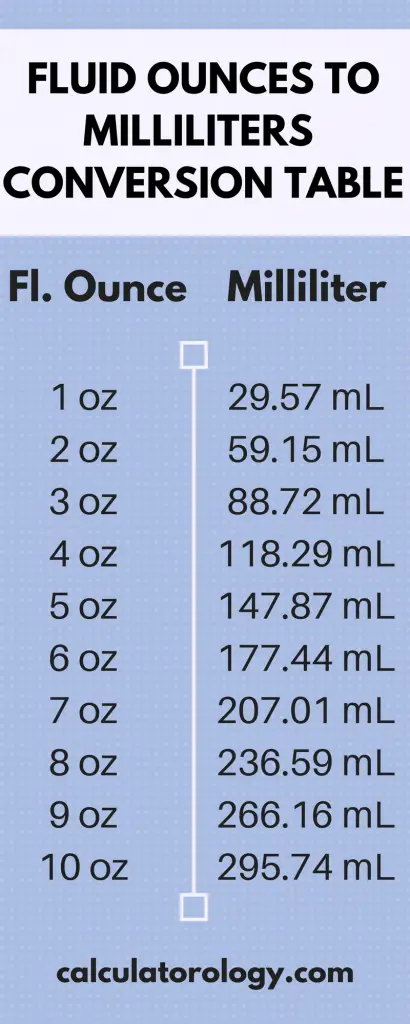код Ounce to Milliliter - Calculatorology

## Ounces to Milliliters Conversion

Converter

Enter a US fluid ounce value that you want to convert into milliliters and click on the "convert" button.

### Ounce to Milliliter (How many milliliters in an ounce?)

Fluid Ounce (oz.) is a US and an imperial customary volume unit. It is a mass (weight0 unit which makes it different from fluid ounce. A milliliter is a volume unit in the metric system abbreviated as "mL" or "ml".

##### Conversions

To determine the number of milliliters available in ounces, we multiply the value by the conversion factor. The converter can be useful if you want to convert from US fluid ounces to milliliters.

1 Fluid Ounce [US] = 29.5735296 Milliliters

1 Fluid Ounce [UK] = 28.4130625 Milliliters

For example, if you want to find the number of milliliters in a half fluid ounce, we multiply the value in fluid ounce by the conversion factor. E.g. ½ fl. Oz. x 29.5735296 = 14.8 ml. in a half fluid ounce [US].

The same procedure is used when you want to determine the number of milliliters [UK] in a half fluid ounce. Multiplying the value in fluid ounce by the conversion factor we get;

½ fl. Oz. x 28.4130625 = 14.2 ml. in a half fluid ounce [UK].#### How many ounces are in milliliters?

By definition, one liter is equivalent to the volume of one kilogram of water. The same amount of water equals 33.814 fluid ounces. Hence, there are 29.5735296 Milliliters in an ounce. To determine how many milliliters are in one ounce, we divide the number of milliliters in a liter by the number of fluid ounces in a liter.

The number of milliliters in a liter is 1000 while the number of fluid ounces in a liter is 33.814. This can be expressed as;

1000/33.814 = 29.5735296 ml. in an ounce. [US]

The conversion result will also vary depending on the standard used. If you were converting from the UK standard, the number of fluid ounces in a liter will be 35.195. To determine the number of milliliters in one ounce, we divide the milliliters in a liter by fluid ounces in one liter. It can be expressed as;

1000/35.195 = 28.4130625 ml. in an ounce [UK]

For US fluid ounces, there are approximately 3.7 ml in 1/8 fl. Oz., 21.3 ml in ¾ fl. Oz., 18.9 ml in 2/3 fl. Oz., 9.5 ml in 1/3 fl. oz., and 7.4 ml in a quarter (1/4) fl. Oz.

For UK fluid ounces, there are approximately 3.55 ml in 1/8 fl. Oz, 21.3 ml in ¾ fl. Oz., 18.9 ml in 2/3 fl. oz., 9.5 ml in 1/3 fl. Oz, and 7.1 ml in a quarter (1/4) fl. Oz.